Back

# Forever Functional: Random Unit Testing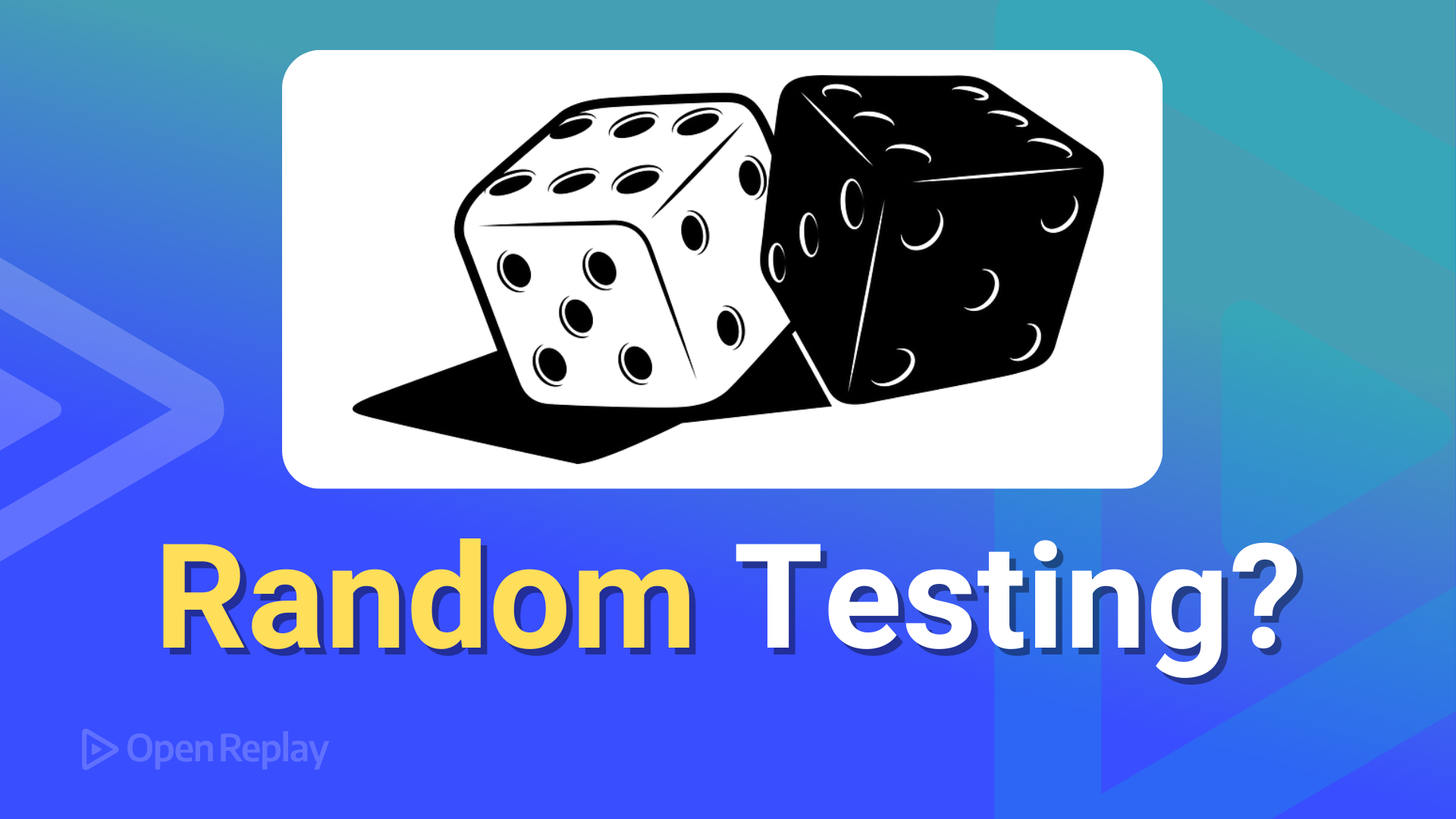Suppose you have a function that produces results based on random numbers. How can you go about writing an unit test for it? And, even more importantly, how can you be sure that your function’s random behavior produces the right results? This article will show you techniques for writing unit tests that answer both questions.Get to the root cause of every issue
Observe user actions for quicker issue troubleshooting with OpenReplay.

Discover how at OpenReplay.com.

## FOREVER FUNCTIONAL — Random Unit Testing

In the previous article, we went over several important Computer Science methods (like shuffling or sampling) that produce results based on random numbers. For example, suppose you want to generate a random card. In that case, you’d want every possible card to be equally probable, and if you have to deal Poker hands, every possible combination of cards should have the same probability of being dealt.

However, this dependency on random numbers has a problem; how would you do unit testing for such methods? Unit testing is based on providing known inputs and validating that the tested function returns the expected results. However, in our case, we expect different results every time! So, what can we do?

In this article, we’ll base our examples on two game-related topics: the Craps dice game, based on rolling two dice, and shuffling a deck of cards, needed for many games. We’ll see how to write tests for these functions, despite the inherent randomness in their output. And, as an extra value, an anecdote: this kind of testing saved me from publishing the wrong shuffling code, so it’s worth it!

## Solution (?) #1: Ignore the problem (AKA “Hope for the best”)

Let’s begin with a non-starter: what about not doing any testing? You could run the code several times, check that the output looks random enough, and be done with it! If you think this is wise, you should give a look at this article, How We Learned to Cheat at Online Poker: A Study in Software Security, which led to finding an error in the shuffling logic that led to the investigators being able to know what cards were held by each player, and what cards would be coming up; in short, everything they needed to thoroughly beat everybody else!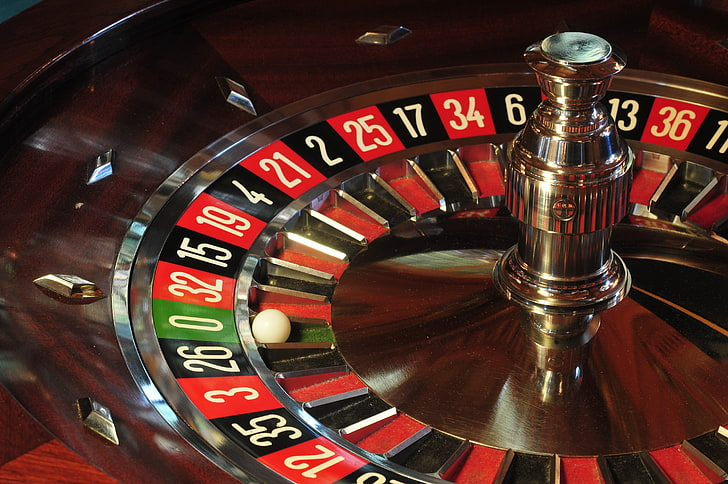By the way, non-randomness is not only a problem with computer algorithms; a non-random roulette wheel can bring huge gains (or losses!) to a Casino. For example, this analysis for a specific case, found out that there was ”substantial evidence that the wheel produced a non-random frequency of occurrence of the numbers […] centered round the numbers 12, 20, 30, and 2 or 21”. These results showed that a ”player selecting [12, 20, 30, and 21] each spin would have won on 12.3% of spins as against the 10.8% expected for a random wheel.” In this case, we are talking about actual physical tests, not unit tests running on a computer. Still, the conclusion is the same: you need to be sure that your random results are actually random.

## Solution #2: Mock randomness

We’ll be showing examples based on the Craps game, so let’s start by writing a function to simulate a random die throw.

``````const randomDie = (): number => {
return 1 + Math.floor(6 * Math.random());
};``````

Math.random() produces a number strictly between 0 and 1, so multiplying it by 6 produces a number between 0 and 6 (but never equal to 6), truncating it produces a 0 to 5 value, and adding 1 generates a 1 to 6 value, as in a die. How can we test this?

For cryptographic purposes, `Math.random()` is not good enough; you should then go for the Crypto `getRandomValues()` API method. Because it’s better known, we’ll keep using `Math.random()`; changing from one method to the other is trivial.

This `randomDie()` function is essentially impure; it will always return different values (that’s what we expect, after all!), so we cannot directly write unit tests for it as is.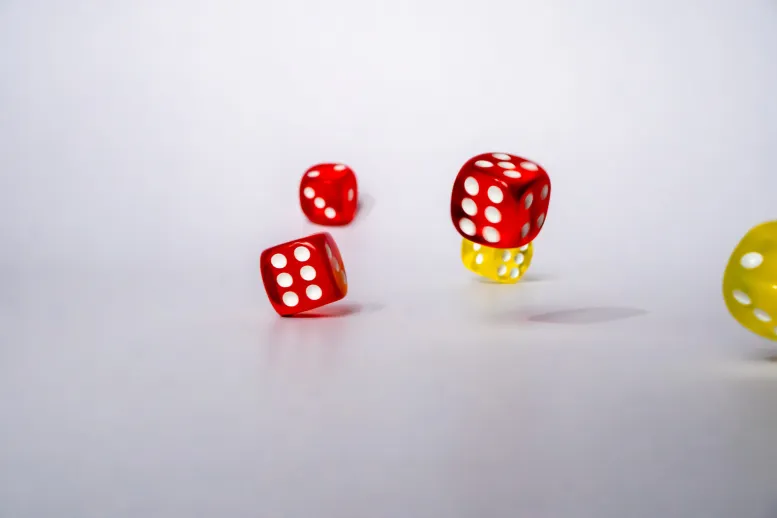A possible solution we’ve already seen in previous articles is making the function less impure. We could write an alternative version that takes an optional parameter for generating random numbers, a `getRandomNum()` function.

``````const randomDie2 = (getRandomNum = () => Math.random()): number => {
return 1 + Math.floor(6 * getRandomNum());
};``````

Suppose we do not provide any argument to `randomDie2()`. In that case, it will work exactly as `randomDie()`, but we can provide a mock implementation of our own for testing purposes, as shown below.

``````describe('Values from randomDie2', () => {
test('should be low for low randoms', () => {
expect(randomDie2(() => 0.01)).toBe(1);
});

test('should be middle for middle randoms', () => {
expect(randomDie2(() => 0.49)).toBe(3);
});

test('should be high for high randoms', () => {
expect(randomDie2(() => 0.99)).toBe(6);
});
});``````

These Jest tests pass, so at least we know that the produced “throws” are according to our expectations; low random numbers produce a 1, high random numbers produce a 6, etc.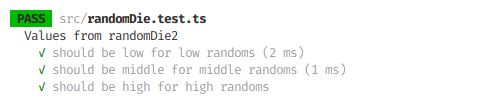You could also do this kind of test by working with Jest mocks. I’m joining both “extreme” tests just for variety to show how to mock a sequence of returned values.

``````describe('Values from randomDie with Jest mock', () => {
test('should be extremes for high & low randoms', () => {
jest.spyOn(Math, 'random').mockReturnValueOnce(0.1).mockReturnValue(0.9);
expect(randomDie()).toBe(1);
expect(randomDie()).toBe(6);
jest.spyOn(Math, 'random').mockRestore();
});

test('should be middle for middle randoms', () => {
jest.spyOn(Math, 'random').mockReturnValueOnce(0.49);
expect(randomDie()).toBe(3);
jest.spyOn(Math, 'random').mockRestore();
});
});``````

And should you not want to directly mess with the `Math` object, you could go with the jest-mock-random library and write instead:

``````describe('Values from randomDie with random-mock', () => {
test('should be extremes for high & low randoms', () => {
mockRandom([0.1, 0.9]);
expect(randomDie()).toBe(1);
expect(randomDie()).toBe(6);
resetMockRandom();
});

test('should be middle for middle randoms', () => {
mockRandom([0.49]);
expect(randomDie()).toBe(3);
resetMockRandom();
});
});``````

OK, we can be sure that the `randomDie()` function returns values from 1 to 6… but is this good enough? Are values always from 1 to 6? Do we get a random sequence? We are assuming that it returns values with the right equal frequencies, but that depends on hoping that the used random number generator is actually random enough. For all we know, it could return low values more often than high ones, and then our “die” would produce more ones and twos than fives and sixes!

There are even some more complications. For our Craps game, we’ll need to simulate throwing a pair of dice and getting their sum, as shown below.

``const randomThrow = (): number => randomDie() + randomDie();``

The result will be a number between 2 and 12. Still, the odds are not the same for all results: a 2 (“Snake Eyes”) result should come up about once for every six “Natural Seven” results. While each single die result has the same probability as any other result, Craps results have different probabilities.

Given the issues above, it’s clear we need different tests; let’s go into some Statistics!

## An interlude: Some Statistics

How do we measure if observed data (a “sample”) matches an expected model? The Chi-squared (χ²) test is a goodness of fit test that measures how a model matches actual data, how likely it is that the difference between those sets is just due to pre chance. It can be used whenever we have random, independent data; it works perfectly well with simulated dice throws or shuffled decks, for instance, and that’s what we need.In more precise terms, Chi-squared tests are used to test hypotheses by measuring the size of the differences between the expected and the actual results. The test needs some calculations to be done and a “significance level” —say, 1%— and if the result is greater than a certain value (that depends on the sample’s size), we can say that the probability of that sample corresponding to the model is lower than 1%, so it’s likely the sample is from a different model. Obviously, there’s always a chance that extreme differences may happen (for instance, a die could produce 100 sixes in a row), but if needed, you can re-run the test; if it again suggests the model isn’t right, the probability of that occurring by pure chance would be 1% of 1%.

How does the Chi-squared test work? Given a sample, we must group them into categories or cells. For instance, for our die simulator, categories could be the numbers from 1 to 6; for our Craps game, numbers from 2 to 12 would work. Let’s be a bit mathematical, and assume:

• n is the number of categories
• Oᵢ is the count of observations of values of type i
• Eᵢ is the expected count of values of type i. We need all Eᵢ to be at least 5; the test won’t give good results for low expected counts.
• N is the total number of observations; it equals the sum of all Oᵢ and also of all Eᵢ

We then calculate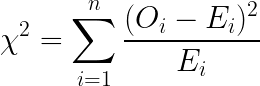or, equivalently,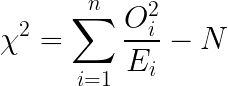Then we check the result with a table, to decide if it’s likely or not that the observations match the model.

(In case you are interested; I created the formulas above at https://editor.codecogs.com/)

The needed table is a complication, but numerical approximation methods work well. The basic code is as follows:

``````function chiSquareAccepted(actual: number[], expected: number[]): boolean {
const deltas = actual.map((v, i) => (actual[i] - expected[i]) ** 2 / expected[i]);
const chi2 = deltas.reduce((x, y) => x + y, 0);

return compute(chi2, actual.length - 1) < 0.999; // We want to be at least 99.9% of the results
}``````

If you wish to use the second formula we saw above, you could change the first two lines to:

`````` const deltas2 = actual.map((v, i) => actual[i] ** 2 / expected[i]);
const count = actual.reduce((x, y) => x + y, 0);
const chi2 = deltas2.reduce((x, y) => x + y, -count);``````

The `actual` array has the counts for the observed data; the `expected` array has the theoretically expected results. We could (should) add some defensive programming and check the following:

• arrays must not be empty
• `actual` and `expected` must be the same length
• the arrays must have at least two elements
• the sum of values in the arrays must be the same
• all array values must be non-negative
• all expected values should be 5 or greater

These tests are easy to write, so I’ll leave them up to you as an exercise! The final part of the function is the tricky one. The `compute()` function is the complex one, that checks if the provided Chi-squared value is above or below the desired probability limits; the version I use is available online, and you can see it at the end of the article.

## Solution #3: Test the output for randomness

OK, given the Chi-squared test we just saw, how do we use it to test for randomness? Let’s first check our single die throw code and the Craps game throw, and then consider the deck shuffling code, which has some extra considerations.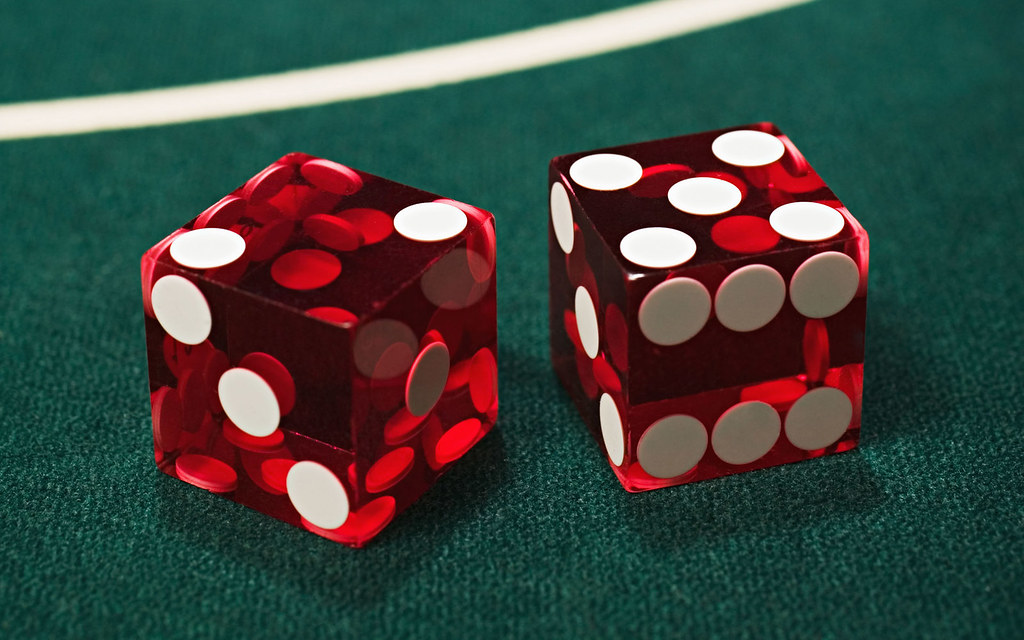### Testing dice throws

Let’s consider our `randomDie()` function; if we run it `TRIES` times, we’d expect all values from 1 to 6 to appear each one `TRIES/6` times. We need expected values to be at least 5 (let’s go with 10 for extra safety), so `TRIES` needs to be 60 or more. We can write a test as follows.

``````describe('Seen values', () => {
test('should match the expected ones', () => {
const TRIES = 1200;
const seen = new Array(6).fill(0);
for (let i = 0; i < TRIES; i++) {
const dieThrow = randomDie();
seen[dieThrow - 1]++;
}
const expected = new Array(6).fill(TRIES / 6);

expect(chiSquareAccepted(seen, expected)).toBeTruthy();
});
});``````

We simulate `TRIES` throws and count the times 1, 2, … 6 come up. (Careful! The count for the 1 result goes in seen, so we have to make an adjustment before counting.) The expected values are just `TRIES/6`; each result should appear 1/6 of the time. Finally, we use our `chiSquareAccepted()` function, which should return `true`. We know that the `randomDie()` function is correct (for mathematical reasons), but anyway, there’s a probability of 1 in a thousand that some test will produce results that don’t look random enough. If you want to be on the safer side, in case the calculation fails, you could run it again; if you get two failures in a row, the code deserves a second careful look!

Let’s see that the test also detects bad functions. We could set up two loaded dice; one will produce a 1 20% of the time, and the other will produce a 6 only 10% of the time. (Proper expected probabilities should be 16.67% for both numbers.) We can write tests and verify those crooked functions are detected.

``````export const badDie1 = (): number =>
Math.random() < 0.2 ? 1 : 2 + Math.floor(5 * Math.random());

export const badDie6 = (): number =>
Math.random() > 0.9 ? 6 : 1 + Math.floor(5 * Math.random());

describe('With crooked dice', () => {
test('both fail', () => {
const TRIES = 1200;
const seen1 = new Array(6).fill(0);
const seen6 = new Array(6).fill(0);
for (let i = 0; i < TRIES; i++) {
}
const expected = new Array(6).fill(TRIES / 6);

expect(chiSquareAccepted(seen1, expected)).toBeFalsy();
expect(chiSquareAccepted(seen6, expected)).toBeFalsy();
});
});``````

Both false dice are detected; the probability of these functions matching an honest die is less than 0.1%.

Let’s finish by testing our Craps game simulation. Results 2 and 12 come up only once in 36 tries, so to get an expected value not smaller than 5, we need at least 180 tries.

``````const randomThrow = (): number => randomDie() + randomDie();

describe('Craps simulation', () => {
test('with honest dice', () => {
const TRIES = 900;

const craps = new Array(11).fill(0);
for (let i = 0; i < TRIES; i++) {
craps[randomDie() - 1 + randomDie() - 1]++;
}

const expected = new Array(11).fill(0);
for (let i = 1; i <= 6; i++) {
for (let j = 1; j <= 6; j++) {
expected[i - 1 + j - 1] += TRIES / 36;
}
}

expect(chiSquareAccepted(craps, expected)).toBeTruthy();
});
});``````

Generating the `craps` array with the seen counts is easy; the `expected` array requires a bit of work to match the actual probabilities of each result.

It should be clear by now that we can use the Chi-squared test very straightforwardly for any kind of random result with a few categories. For instance, you could try out a roulette wheel simulator (37 or 38 possible counts, depending on how many zeroes your wheel has), a random card dealing function (52 possible counts if no jokers are present), etc.; the number of tries must be high enough so all expected values are at least 5, as we mentioned. We’re done! (More or less… we’ll come back to more tests later.)

However… what would happen if the number of expected categories is too large? For instance, what would happen if you wanted to test a Powerball simulator, that has 292,201,338 possible results, or our own deck shuffling code, that can an impossibly high number of different deck orderings? We need to tackle things a bit differently.

### Testing shuffling resultsIn our previous article on shuffling, we showed the result of randomly shuffling a 4-card deck 24,000 times:

``````A-B-C-D:   983 ###############################################
A-B-D-C:  1026 #################################################
A-C-B-D:   977 ##############################################
A-C-D-B:   993 ###############################################
A-D-B-C:   983 ###############################################
A-D-C-B:   984 ###############################################
B-A-C-D:  1028 #################################################
B-A-D-C:   986 ###############################################
B-C-A-D:  1033 #################################################
B-C-D-A:  1016 ################################################
B-D-A-C:   957 ##############################################
B-D-C-A:  1022 #################################################
C-A-B-D:   989 ###############################################
C-A-D-B:   962 ##############################################
C-B-A-D:   993 ###############################################
C-B-D-A:  1028 #################################################
C-D-A-B:   955 #############################################
C-D-B-A:  1004 ################################################
D-A-B-C:  1023 #################################################
D-A-C-B:  1051 ##################################################
D-B-A-C:   985 ###############################################
D-B-C-A:  1020 #################################################
D-C-A-B:   990 ###############################################
D-C-B-A:  1012 ################################################
COUNT= 24``````

Is this result good enough? We can try it out with a single line of code:

``````console.log(
'SHUFFLING',
chiSquareAccepted(
[
983, 1026, 977, 993, 983, 984, 1028, 986, 1033, 1016, 957, 1022, 989, 962,
993, 1028, 955, 1004, 1023, 1051, 985, 1020, 990, 1012
],
new Array(24).fill(1000)
)
);
// Output: true (it's unlikely the results were distributed this way by chance)
``````

However, randomly shuffling a deck can produce 52! (52 factorial) different results, which is more than 80 million million million million million million million million million million million (that’s an 80 followed by 66 zeros) possibilities, so doing the Chi-squared test in a direct way is not possible, at least for almost any deck size.

What can we do? A possible solution is picking one random card and, over many shuffling runs, check if the card has ended in all possible deck positions equally. To simplify our logic, let’s just shuffle a list of numbers.

``````type Deck = number[];

const makeDeck = (size: number): Deck =>
new Array(size).fill(0).map((v, i) => i + 1);

function shuffle(deck: Deck): Deck {
for (let i = deck.length - 1; i > 0; i--) {
const j = randomInt(i + 1);
[deck[i], deck[j]] = [deck[j], deck[i]];
}
return deck;
}``````

Test code could then be:

``````describe('Random shuffling', () => {
test('should distribute cards equally', () => {
const CARDS = 52;
const ROUNDS = 50;
const TRIES = CARDS * ROUNDS;
const seenAt = new Array(52).fill(0);
const card = randomInt(CARDS) + 1;
for (let i = 0; i < TRIES; i++) {
const deck = shuffle(makeDeck(CARDS));
seenAt[deck.indexOf(card)]++;
}
expect(
chiSquareAccepted(seenAt, new Array(CARDS).fill(ROUNDS))
).toBeTruthy();
});
});``````

We do 50 rounds of testing. We pick a random `card`, and in each test we shuffle a new deck and then look for the position of `card` in it. Everything works, though if you try with low values of ROUNDS (greater than 5, obviously, but not too much greater than it), the test may fail to work.

As I wrote earlier, this kind of test helped me avoid a mistake. I had written the shuffling code like this:

``````function shuffle(deck: Deck): Deck {
for (let i = deck.length - 1; i > 0; i--) {
const j = randomInt(i);
[deck[i], deck[j]] = [deck[j], deck[i]];
}
return deck;
}``````

Spot the error? The value of `j` should be a random number between 0 and `i` inclusive, but the way `randomInt()` works, we actually get a value between 0 and `i-1`. When I ran tests for this (wrong) logic, they did fail, which led me to review all the code and finally spot the issue; a plus for the test!

## Further considerations

We have been seeing tests for randomness, but you should be aware that this is not enough. For instance, a “random” die throw function that returned 1, 2, 3, 4, 5, 6, 1, 2, 3, 4, 5, 6, 1, 2 … would be accepted (because it produces all possible results with equal counts) but it’s certainly not random!To better check for randomness, you must also include some other kinds of tests. A possible test measures autocorrelation: pick a value of k>1, and repeatedly generate k random values in succession, applying the Chi-squared test to the distribution of the pairs formed by the first and last values. To make this clearer, suppose you were testing a coin toss simulator. With k=2, you’d be essentially checking if all possible pairs of results (Head/Head, Head/Tails, Tails/Head, Tails/Tails) are equally produced. If you applied this autocorrelation test to the sequential die function in the previous paragraph, it would fail because not all possible pairs would be produced.

You can do even better; you could do autocorrelation tests with three or four values instead of just two. The possible problem is that the universe of expected combinations might grow too much.

There are yet more possible tests: the Wald-Wolfowitz Runs Test is a possibility. It essentially looks at the sequence of produced values, and calculates the probability of the sequence actually being random. The bad random die sequence at the end of the last paragraph would be detected as not likely to actually being random, for instance.

## Conclusion

Testing random-based functions is not trivial, but statistical methods help us here. Working with the Chi-squared test (an alternative may be Fisher’s Exact Test; check it out), we were able to produce unit tests that allow us to estimate if the generated random results match or not an expected distribution.

Unit tests are always expected to pass, but here, since we’re dealing with probabilities, there’s still a certain chance that a test will fail, despite being applied to a correct function. We have suggested a way out of this: automatically re-running a test in case of a failure, drastically diminishing the probability of a false error.

## Appendix: Chi-squared calculations

The following code is taken from https://www.math.ucla.edu/~tom/distributions/chisq.html; I just adapted it to modern TypeScript code.

``````function LogGamma(Z: number): number {
const S =
1 +
76.18009173 / Z -
86.50532033 / (Z + 1) +
24.01409822 / (Z + 2) -
1.231739516 / (Z + 3) +
0.00120858003 / (Z + 4) -
0.00000536382 / (Z + 5);
const LG =
(Z - 0.5) * Math.log(Z + 4.5) - (Z + 4.5) + Math.log(S * 2.50662827465);
return LG;
}

function Gcf(X: number, A: number): number {
// Good for X>A+1
let A0 = 0;
let B0 = 1;
let A1 = 1;
let B1 = X;
let AOLD = 0;
let N = 0;
while (Math.abs((A1 - AOLD) / A1) > 0.00001) {
AOLD = A1;
N = N + 1;
A0 = A1 + (N - A) * A0;
B0 = B1 + (N - A) * B0;
A1 = X * A0 + N * A1;
B1 = X * B0 + N * B1;
A0 = A0 / B1;
B0 = B0 / B1;
A1 = A1 / B1;
B1 = 1;
}
const Prob = Math.exp(A * Math.log(X) - X - LogGamma(A)) * A1;
return 1 - Prob;
}

function Gser(X: number, A: number): number {
// Good for X<A+1.
let T9 = 1 / A;
let G = T9;
let I = 1;
while (T9 > G * 0.00001) {
T9 = (T9 * X) / (A + I);
G = G + T9;
I = I + 1;
}
G = G * Math.exp(A * Math.log(X) - X - LogGamma(A));
return G;
}

function Gammacdf(x: number, a: number): number {
let GI;
if (x <= 0) {
GI = 0;
} else if (x < a + 1) {
GI = Gser(x, a);
} else {
GI = Gcf(x, a);
}
return GI;
}

function compute(Z: number, DF: number): number {
if (DF <= 0) {
throw new Error('Degrees of freedom must be positive');
}
if (Z < 0) {
throw new Error('X² cannot be negative');
}
const Chisqcdf = Gammacdf(Z / 2, DF / 2);
return Math.round(Chisqcdf * 100_000) / 100_000;
}``````

### Understand every bug

Uncover frustrations, understand bugs and fix slowdowns like never before with OpenReplay — the open-source session replay tool for developers. Self-host it in minutes, and have complete control over your customer data. Check our GitHub repo and join the thousands of developers in our community.